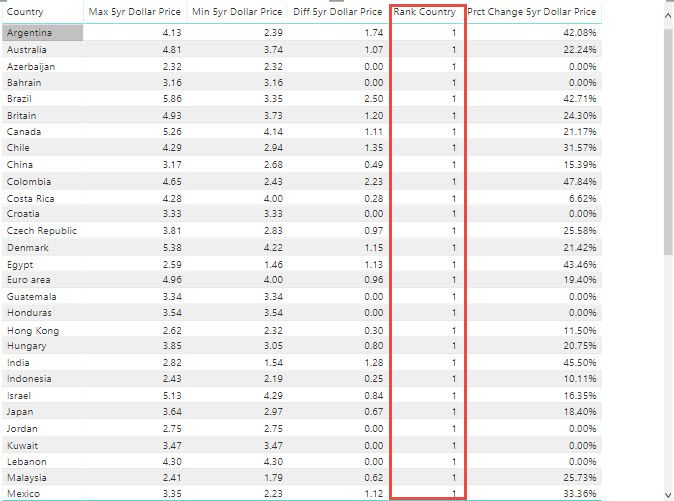cancel
Showing results for
Did you mean:Anonymous
Not applicable

## Ranking based on % measurment

Hello All,

I have created a grid below, with follwing measures

Max 5yr Dollar Price = MAX(DOLLAR_PRICE)
Min 5yr Dollar Price = Min(DOLLAR_PRICE)
Diff 5yr Dollar Price = MAX(DOLLAR_PRICE) -  Min(DOLLAR_PRICE)
Prct Change 5y Dollar Price = MAX(DOLLAR_PRICE) -  Min(DOLLAR_PRICE) / MAX(DOLLAR_PRICE)

I need to rank countries based on Prct Change 5y Dollar Price

Formula I used for Prct Change 5y Dollar Price =

Rank Country = RANKX(ALL('TableName'), [Prct Change 5yr Dollar Price] , , DESC)
Above Formula gices me 1 for every row1 ACCEPTED SOLUTIONSuper User

I would make a couple of changes to your measures:

```Max 5yr Dollar Price = MAX([DOLLAR_PRICE])

Min 5yr Dollar Price = Min([DOLLAR_PRICE])

Diff 5yr Dollar Price = [Max 5yr Dollar Price] -  [Min 5yr Dollar Price]

Prct Change 5y Dollar Price = DIVIDE(([Max 5yr Dollar Price] -  [Min 5yr Dollar Price]),[Max 5yr Dollar Price],0)

Rank =
RANKX(ALL('Table24'[Country]),[Prct Change 5y Dollar Price])```

All of those are just coding practice changes except for the RANKX.

Become an expert!: Enterprise DNA
External Tools: MSHGQM
Latest book!:
Mastering Power BI 2nd EditionDAX is easy, CALCULATE makes DAX hard...
2 REPLIES 2Super User

I would make a couple of changes to your measures:

```Max 5yr Dollar Price = MAX([DOLLAR_PRICE])

Min 5yr Dollar Price = Min([DOLLAR_PRICE])

Diff 5yr Dollar Price = [Max 5yr Dollar Price] -  [Min 5yr Dollar Price]

Prct Change 5y Dollar Price = DIVIDE(([Max 5yr Dollar Price] -  [Min 5yr Dollar Price]),[Max 5yr Dollar Price],0)

Rank =
RANKX(ALL('Table24'[Country]),[Prct Change 5y Dollar Price])```

All of those are just coding practice changes except for the RANKX.

Become an expert!: Enterprise DNA
External Tools: MSHGQM
Latest book!:
Mastering Power BI 2nd EditionDAX is easy, CALCULATE makes DAX hard...Anonymous
Not applicable

@Greg_Deckleryou are the man!